Abstract

# ◆ ◆ ◆

1、动机与目标读者

1. LR模型原理简单，并且有一个现成的叫LIBLINEAR 的工具库，易于上手，并且效果不错。
2. LR可以说是互联网上最常用也是最有影响力的分类算法。LR几乎是所有广告系统中和推荐系统中点击率（Click    Through    Rate（CTR））预估模型的基本算法。
3. LR同时也是现在炙手可热的“深度学习”（Deep    Lerning）的基本组成单元，扎实的掌握LR也将有助于你的学好深度学习。

• 了解LR：了解LR模型、L1和L2规则化（Regularization）、为什么L1规则化更倾向于产生稀疏模型（Sparse    Model）、以及稀疏模型的优点。
• 理解LR：理解LR模型的学习算法、能够独自推导基于L-BFGS的L1和L2规则化的LR算法，并将其在MPI平台上并行化实现。
• 改进LR：能够在实际中自如应用LR，持续改进LR来解决实际中未曾遇见到的问题。例如，当数据中的正样本远远小于负样本的情况下（例如，广告点击率预告问题），该怎么调整LR？当数据中有相当部分缺失时该如何调整算法？

1. 高阶机器学习人员：硕士毕业后5年及以上的机器学习经历，你就把这个当成一个关于LR和无约束优化算法的回顾材料好了，如有错误和不足请加以斧正。
2. 中阶机器学习人员：硕士毕业后3~5年的机器学习经历，你可以把这个当做学习资料，把以前学过的东西串在一起，查漏补缺，做到真正懂得LR和相关优化的算法，从而能对工程实践做出正确的指导。
4. 机器学习人员以外的高富帅和白富美们：你只需要知道LR是一个好用的自动分类算法，吩咐研究猿和攻城狮做就好了。另外，还可以用这篇文章嘲弄机器学习的屌丝们：累死累活，死那么多脑细胞，挣那两儿钱。

# *鉴于文章的长度，这里只对文章内容做标题概览

2、初识逻辑回归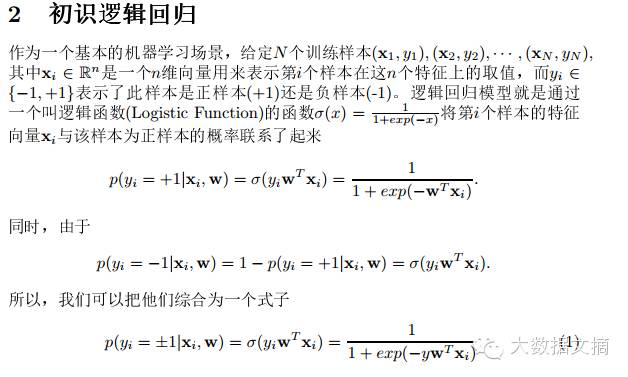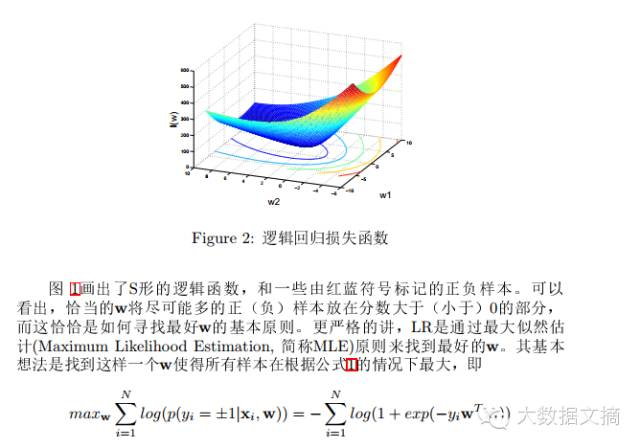3、L1    vs.    L2规则化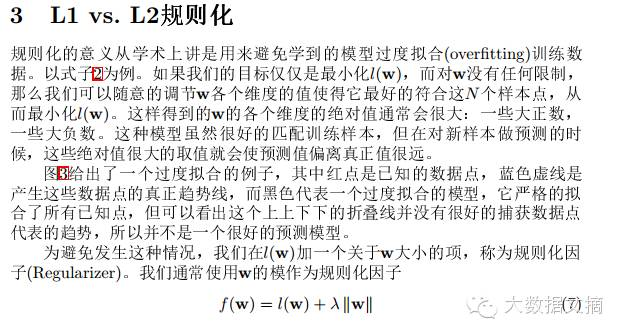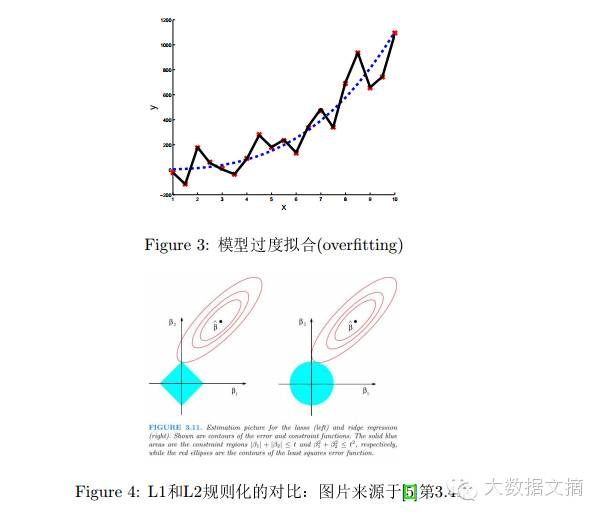4、求解L2规则化的逻辑回归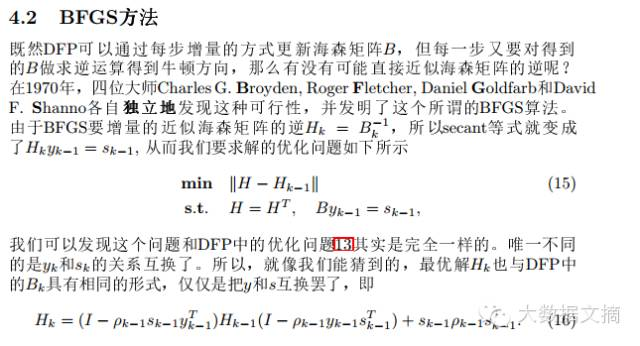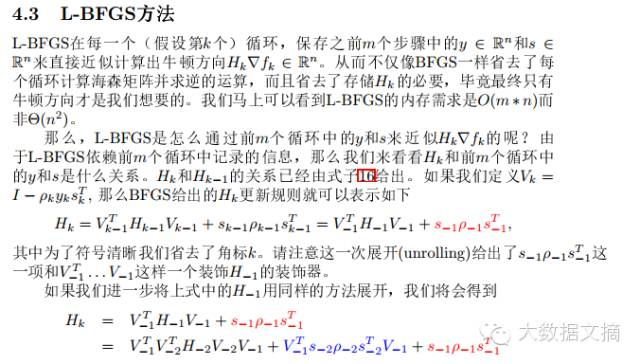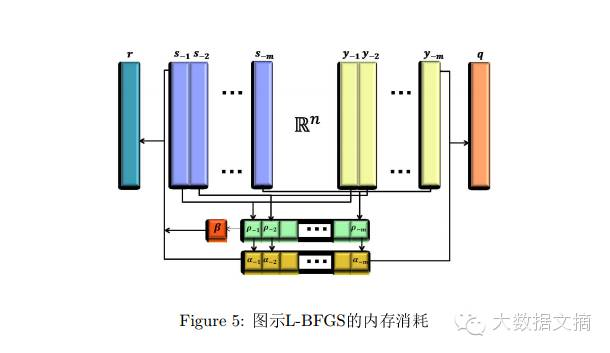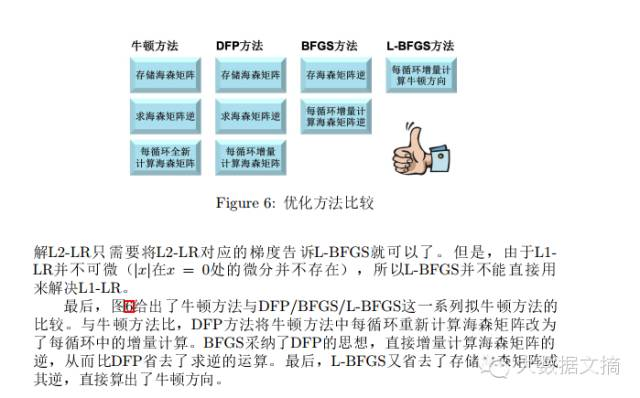5、OWL-QN：用L-BFGS求解L1规则化的逻辑回归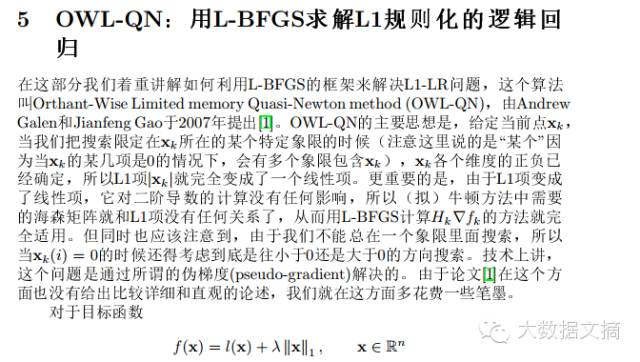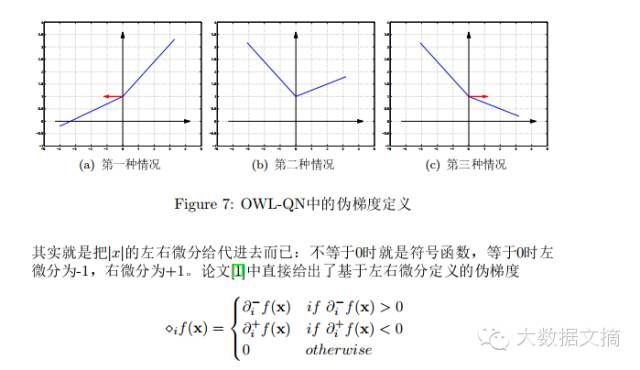6、相关工作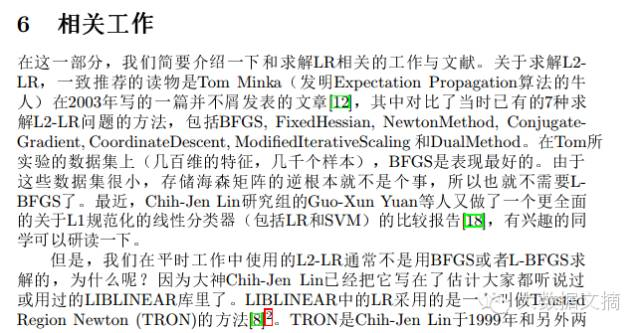7、前沿研究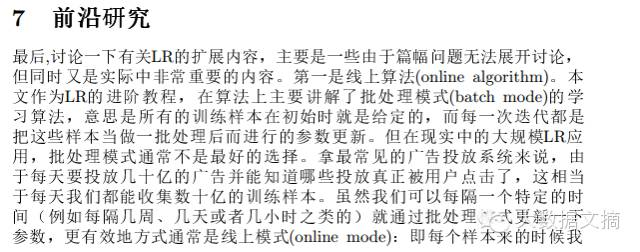# ◆ ◆ ◆

2012年，柳超博士回国加入腾讯科技（北京）有限公司，领导“腾讯搜索”的相关数据挖掘与机器学习业务。2014年，在腾讯与搜狗的战略合并之际加入搜狗科技，出任首席科学家，从零组建了搜狗数据科学研究院，全面负责搜狗互联网业务的数据挖掘与机器学习的前沿研究。

## 算法与算法工程师，技术与技术人员

（注：标题里的算法，指机器学习算法，或者说“算法工程师”这个职位名称里的“算法”，不是“算法与数据结构”里的那个算法。谁能告诉我有没有什么更好的名字来区别这它们，或许是“机器学习算法”与“传统算法”？）

《代码大全》里有个比喻，如果你的问题是给自己的爱犬造一个小窝，那么动手做就是了，如果出了什么错误，大不了重做一个，最多浪费一下午的时间。而造一个摩天大楼就不同了。所以，如果写一份“狗窝”级别的程序，算法、数据结构、设计模式这些又有多大意义呢？甚至违反了DRY原则也没关系，反正一段代码也拷贝不了几次，出了bug就改，大不了重写一次，最多浪费一下午。而且，说不定这个项目两周之后就被砍掉了。如果你做的是一份“造狗窝”的工作，就算你有造摩天大楼的技术，和屠龙之技又有什么分别呢？唯一的“好处”就是你会据此向老板提出更多的加薪要求，以至于老板对你“另眼相看”。

## 既懂数学又会编程，全方位解读如何成为一名投行Quant工

2016-04-12 UniCareerQuant是做什么的？

Quant的工作就是设计并实现金融的数学模型（主要采用计算机编程），包括衍生物定价，风险估价或预测市场行为等。所以Quant更多可看为工程师，按中国的习惯性分类方法就是理工类人才，而不是文科人才，这个和金融有一定的区别(当然金融也有很多理工的内容)。

(1) Desk Quant

Desk Quant 开发直接被交易员使用的价格模型，优势是接近交易中所遇到的Money和机会，劣势是压力很大。

(2) Model Validating Quant

Model Validating Quant 独立开发价格模型，不过是为了确定Desk Quant开发的模型的正确性。优势是更轻松，压力比较小，劣势是这种小组会比较没有作为而且远离Money。

(3) Research Quant

Research Quant 尝试发明新的价格公式和模型，有时还会执行Blue-Sky Research(不太清楚是什么)，优势是比较有趣(对喜欢这些人来说)，而且你学到很多东西。劣势是有时会比较难证明有你这个人存在(跟科学家一样，没有什么大的成果就没人注意你)

(4) Quant Developer

(5) Statistical Arbitrage Quant

Statistical Arbitrage Quant 在数据中寻找自动交易系统的模式(就是套利系统)，这种技术比起衍生物定价的技术有很大的不同，它主要用在对冲基金里，而且这种位置的回报是极不稳定的。

(6) Capital Quant

Capital Quant 建立银行的信用和资本模型，相比衍生物定价相关的工作，它没有那么吸引人，但是随着巴塞尔II银行协议的到来，它变的越来越重要，你会得到不错的收入(但不会很多)，更少的压力和更少的工作时间。

Quant工作的领域？

(1) FX

FX就是外汇交易的简写。合同趋向于短期，大量的金额和简单的规定，所以重点在于很快速度的建立模型。

(2) Equities

Equities的意思是股票和指数的期权，技术偏向于偏微分方程(PDE)。它并不是一个特别大的市场。

(3) Fixed Income

Fixed Income的意思是基于利息的衍生物，这从市值上来说可能是最大的市场，他用到的数学会更加复杂因为从根本上来说他是多维的，技术上的技巧会用的很多，他的收入比较高。

(4) Credit Derivatives

Credit Derivatives是建立在那些公司债务还清上的衍生产品，他发展的非常快并有大量需求，所以也有很高的收入，尽管如此，他表明了一些当前经济的泡沫因素。

(5) Commodities

Commodities因为最近几年生活用品价格的普遍涨价，也成为一个发展迅速的领域。

(6) Hybrids

Hybrids是多于一个市场的衍生物市场，典型情况是利息率加上一些其它东西，它主要的优势在于可以学到多种领域的知识，这也是当前非常流行的领域。

Quant一般在哪些公司工作？

(1) 商业银行 (HSBC, RBS)

(2) 投行 (高盛, Lehman Brothers)

(4) 会计公司

(5) 软件公司

UniCareer诚意推荐书单

《Options Future and Other Derivatives》
John C. Hull

《Stochastic Calculus for Finance II》
Steven E. Shreve

Shreve的新书，非常elegant， 非常仔细，非常数学完备，适合数学背景， 但是比较厚，对于入门来说还是3好。作者现在CMU纽约。教授。顶尖人物。I是讲离散模型，II讲连续模型。

《Liar’s Poker》
Michael Lewis

《C++ Design Patterns and Derivatives Pricing》
Mark S. Joshi

《Modeling Derivatives in C++ (Wiley Finance)》
Justin London

《The Concepts and Practice of Mathematical Finance》
Mark S. Joshi

《Interest Rate Models – Theory and Practice》
Damiano Brigo / Fabio Mercurio

《Probability with Martingales》
David Williams

《Monte Carlo Methods in Financial Engineering》
Paul Glasserman

《My Life as a Quant: Reflections on Physics and Finance》
Emanuel Derman

1. 关注公众号：UniCareer2. 回复关键字：我爱读书

3. 按照提示完成操作

3个工作日内，Quant十本书福利就会送到你的邮箱啦~## 纯粹数学的雪崩效应：庞加莱猜想何以造福了精准医疗？

2016-04-12 顾险峰 赛先生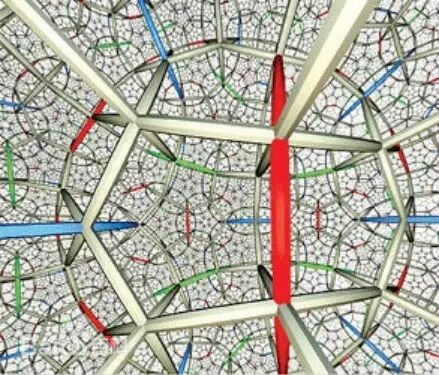1  庞加莱猜想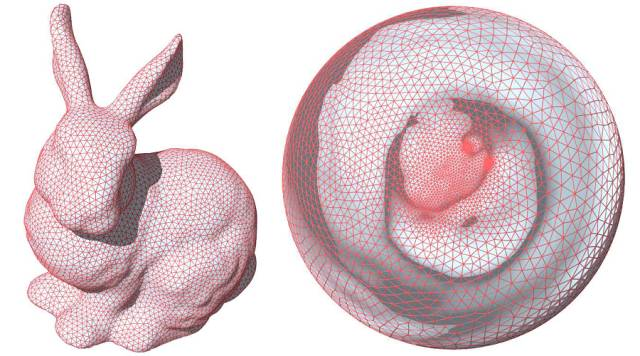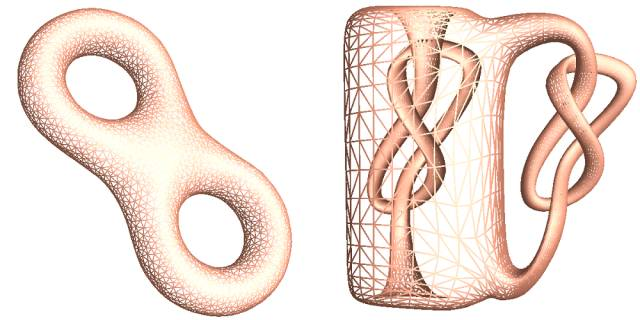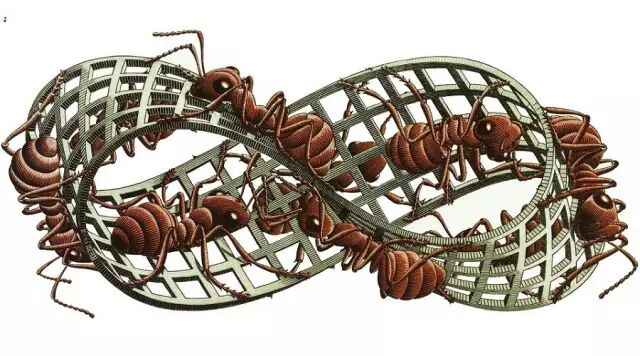图4. 曲面上生活的蚂蚁如何检测曲面的拓扑？2  曲面单值化定理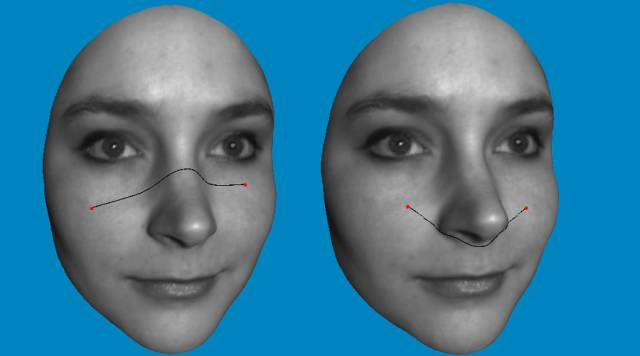3  瑟斯顿几何化猜想4  哈密尔顿的里奇曲率5 庞加莱猜想带来的计算技术6 精准医疗图14. 用里奇曲率流将直肠曲面摊开展平。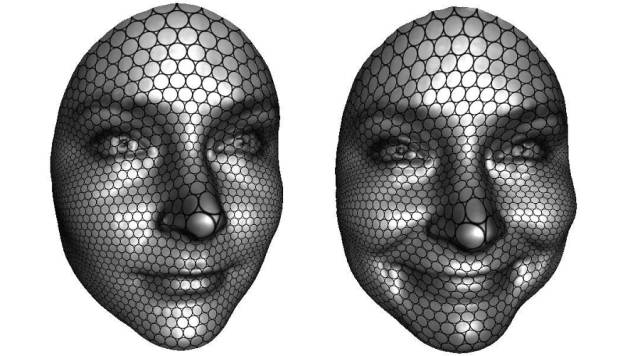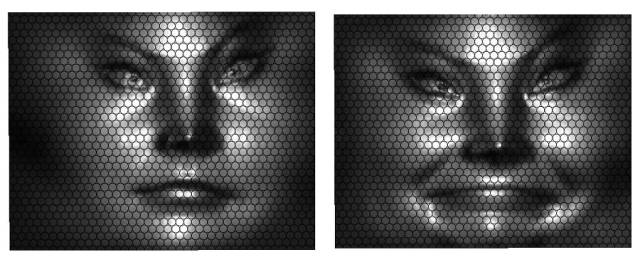7 总结和展望

（如果读者对于离散曲面里奇流的理论、算法和应用有兴趣，可以进一步查阅专著【1】）

 W. Zeng and X. Gu, Ricci Flow for Shape Analysis and Surface Registration Theories, Algorithms and Applications, Springer 2013

如果没有基础科学，我们将会失去什么？②  视频 | 拓扑为何？

## 机器学习算法一览（附python和R代码）

2016-04-19 大数据文摘

—— 埃里克 施密特（谷歌首席执行官）

# ◆ ◆ ◆

## 常见的机器学习算法

`1.线性回归 (Linear Regression) 2.逻辑回归 (Logistic Regression) 3.决策树 (Decision Tree) 4.支持向量机（SVM） 5.朴素贝叶斯 (Naive Bayes) 6.K邻近算法（KNN） 7.K-均值算法（K-means） 8.随机森林 (Random Forest) 9.降低维度算法（Dimensionality Reduction Algorithms） 10.Gradient Boost和Adaboost算法`

### 1.线性回归 (Linear Regression)

• Y- 因变量
• a- 斜率
• X- 自变量
• b- 截距

a和b可以通过最小化因变量误差的平方和得到（最小二乘法）。Python 代码

1. `#Import Library`
2. `#Import other necessary libraries like pandas, numpy...`
3. `from sklearn import linear_model`
4. `#Load Train and Test datasets`
5. `#Identify feature and response variable(s) and values must be numeric and numpy arrays`
6. `x_train=input_variables_values_training_datasets`
7. `y_train=target_variables_values_training_datasets`
8. `x_test=input_variables_values_test_datasets`
9. `# Create linear regression object`
10. `linear = linear_model.LinearRegression()`
11. `# Train the model using the training sets and check score`
12. `linear.fit(x_train, y_train)`
13. `linear.score(x_train, y_train)`
14. `#Equation coefficient and Intercept`
15. `print('Coefficient: \n', linear.coef_)`
16. `print('Intercept: \n', linear.intercept_)`
17. `#Predict Output`
18. `predicted= linear.predict(x_test)`

R 代码

1. `#Load Train and Test datasets`
2. `#Identify feature and response variable(s) and values must be numeric and numpy arrays`
3. `x_train <- input_variables_values_training_datasets`
4. `y_train <- target_variables_values_training_datasets`
5. `x_test <- input_variables_values_test_datasets`
6. `x <- cbind(x_train,y_train)`
7. `# Train the model using the training sets and check score`
8. `linear <- lm(y_train ~ ., data = x)`
9. `summary(linear)`
10. `#Predict Output`
11. `predicted= predict(linear,x_test) `

### 2.逻辑回归

`odds= p/ (1-p) = probability of event occurrence / probability of not event occurrence ln(odds) = ln(p/(1-p)) logit(p) = ln(p/(1-p)) = b0+b1X1+b2X2+b3X3....+bkXk`Python 代码

1. `#Import Library`
2. `from sklearn.linear_model import LogisticRegression`
3. `#Assumed you have, X (predictor) and Y (target) for training data set and x_test(predictor) of test_dataset`
4. `# Create logistic regression object`
5. `model = LogisticRegression()`
6. `# Train the model using the training sets and check score`
7. `model.fit(X, y)`
8. `model.score(X, y)`
9. `#Equation coefficient and Intercept`
10. `print('Coefficient: \n', model.coef_)`
11. `print('Intercept: \n', model.intercept_)`
12. `#Predict Output`
13. `predicted= model.predict(x_test)`

R 代码

1. `x <- cbind(x_train,y_train)`
2. `# Train the model using the training sets and check score`
3. `logistic <- glm(y_train ~ ., data = x,family='binomial')`
4. `summary(logistic)`
5. `#Predict Output`
6. `predicted= predict(logistic,x_test)`

• 加入交互项（interaction）
• 减少特征变量
• 正则化（regularization
• 使用非线性模型

### 3.决策树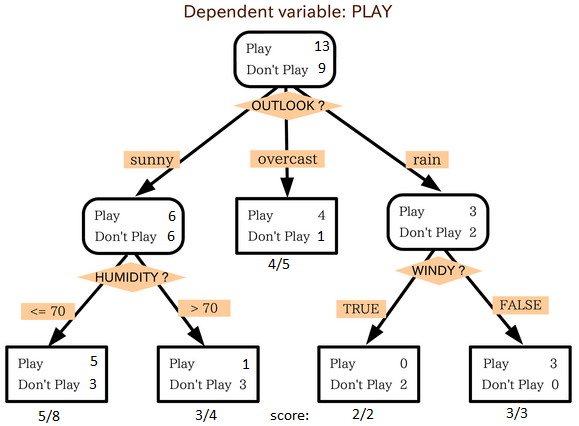Python 代码

1. `#Import Library`
2. `#Import other necessary libraries like pandas, numpy...`
3. `from sklearn import tree`
4. `#Assumed you have, X (predictor) and Y (target) for training data set and x_test(predictor) of test_dataset`
5. `# Create tree object `
6. `model = tree.DecisionTreeClassifier(criterion='gini') # for classification, here you can change the algorithm as gini or entropy (information gain) by default it is gini  `
7. `# model = tree.DecisionTreeRegressor() for regression`
8. `# Train the model using the training sets and check score`
9. `model.fit(X, y)`
10. `model.score(X, y)`
11. `#Predict Output`
12. `predicted= model.predict(x_test)`

R 代码

1. `library(rpart)`
2. `x <- cbind(x_train,y_train)`
3. `# grow tree `
4. `fit <- rpart(y_train ~ ., data = x,method="class")`
5. `summary(fit)`
6. `#Predict Output `
7. `predicted= predict(fit,x_test)`

### 4. 支持向量机（SVM）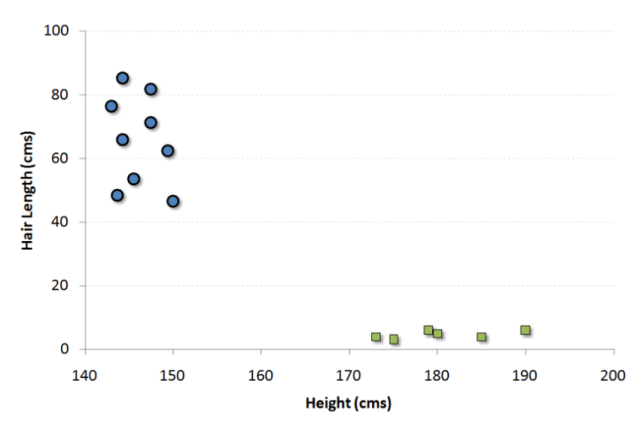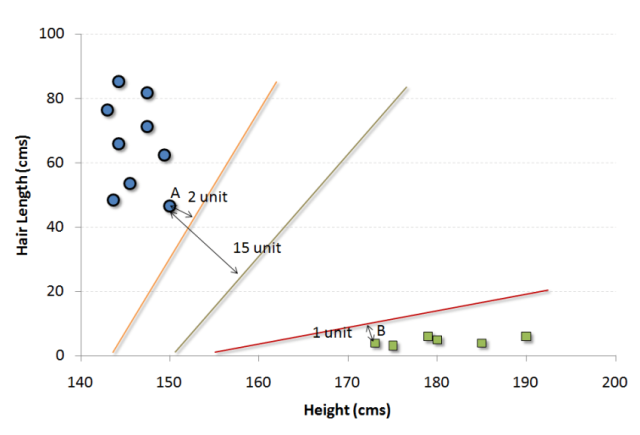#### 我们可以把这个算法想成n维空间里的JezzBall游戏，不过有一些变动：

• 你可以以任何角度画分割线/分割面（经典游戏中只有垂直和水平方向）。
• 现在这个游戏的目的是把不同颜色的小球分到不同空间里。
• 小球是不动的。

Python 代码

1. `#Import Library`
2. `from sklearn import svm`
3. `#Assumed you have, X (predictor) and Y (target) for training data set and x_test(predictor) of test_dataset`
4. `# Create SVM classification object `
5. `model = svm.svc() # there is various option associated with it, this is simple for classification. You can refer link, for mo# re detail.`
6. `# Train the model using the training sets and check score`
7. `model.fit(X, y)`
8. `model.score(X, y)`
9. `#Predict Output`
10. `predicted= model.predict(x_test)`

R 代码

1. `library(e1071)`
2. `x <- cbind(x_train,y_train)`
3. `# Fitting model`
4. `fit <-svm(y_train ~ ., data = x)`
5. `summary(fit)`
6. `#Predict Output `
7. `predicted= predict(fit,x_test)`

### 5. 朴素贝叶斯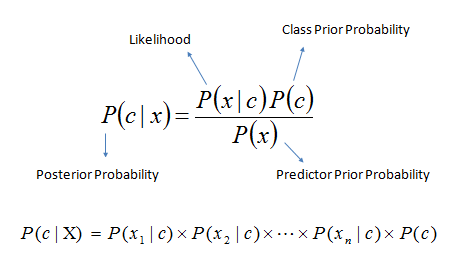• P(c|x)是已知特征x而分类为c的后验概率。
• P(c)是种类c的先验概率。
• P(x|c)是种类c具有特征x的可能性。
• P(x)是特征x的先验概率。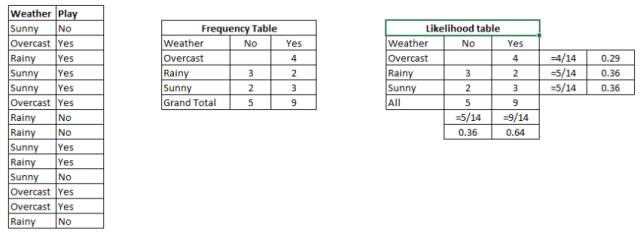Python 代码

1. `#Import Library`
2. `from sklearn.naive_bayes import GaussianNB`
3. `#Assumed you have, X (predictor) and Y (target) for training data set and x_test(predictor) of test_dataset`
4. `# Create SVM classification object model = GaussianNB() # there is other distribution for multinomial classes like Bernoulli Naive Bayes, Refer link`
5. `# Train the model using the training sets and check score`
6. `model.fit(X, y)`
7. `#Predict Output`
8. `predicted= model.predict(x_test)`

R 代码

1. `library(e1071)`
2. `x <- cbind(x_train,y_train)`
3. `# Fitting model`
4. `fit <-naiveBayes(y_train ~ ., data = x)`
5. `summary(fit)`
6. `#Predict Output `
7. `predicted= predict(fit,x_test)`

### 6.KNN（K-邻近算法）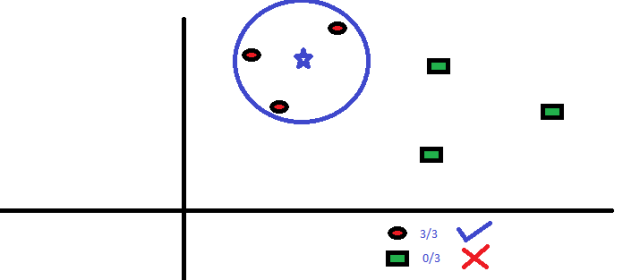KNN在生活中的运用很多。比如，如果你想了解一个不认识的人，你可能就会从这个人的好朋友和圈子中了解他的信息。

• KNN的计算成本很高
• 所有特征应该标准化数量级，否则数量级大的特征在计算距离上会有偏移。
• 在进行KNN前预处理数据，例如去除异常值，噪音等。

Python 代码

1. `#Import Library`
2. `from sklearn.neighbors import KNeighborsClassifier`
3. `#Assumed you have, X (predictor) and Y (target) for training data set and x_test(predictor) of test_dataset`
4. `# Create KNeighbors classifier object model `
5. `KNeighborsClassifier(n_neighbors=6) # default value for n_neighbors is 5`
6. `# Train the model using the training sets and check score`
7. `model.fit(X, y)`
8. `#Predict Output`
9. `predicted= model.predict(x_test)`

R 代码

1. `library(knn)`
2. `x <- cbind(x_train,y_train)`
3. `# Fitting model`
4. `fit <-knn(y_train ~ ., data = x,k=5)`
5. `summary(fit)`
6. `#Predict Output `
7. `predicted= predict(fit,x_test)`

### 7. K均值算法（K-Means）K均值算法如何划分集群：

1. 从每个集群中选取K个数据点作为质心（centroids）。
2. 将每一个数据点与距离自己最近的质心划分在同一集群，即生成K个新集群。
3. 找出新集群的质心，这样就有了新的质心。
4. 重复2和3，直到结果收敛，即不再有新的质心出现。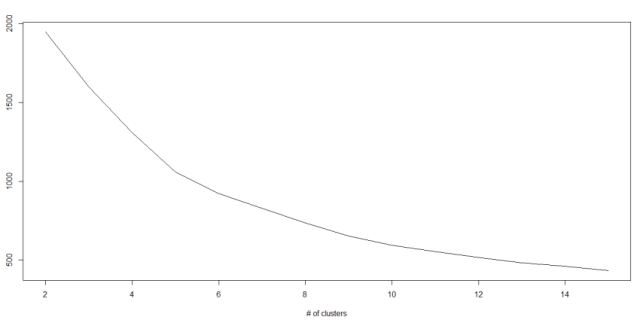Python 代码

1. `#Import Library`
2. `from sklearn.cluster import KMeans`
3. `#Assumed you have, X (attributes) for training data set and x_test(attributes) of test_dataset`
4. `# Create KNeighbors classifier object model `
5. `k_means = KMeans(n_clusters=3, random_state=0)`
6. `# Train the model using the training sets and check score`
7. `model.fit(X)`
8. `#Predict Output`
9. `predicted= model.predict(x_test)`

R 代码

1. `library(cluster)`
2. `fit <- kmeans(X, 3) # 5 cluster solution`

### 8.随机森林

1. 如果训练集中有N种类别，则有重复地随机选取N个样本。这些样本将组成培养决策树的训练集。
2. 如果有M个特征变量，那么选取数m << M，从而在每个节点上随机选取m个特征变量来分割该节点。m在整个森林养成中保持不变。
3. 每个决策树都最大程度上进行分割，没有剪枝。

1. Introduction to Random forest – Simplified
2. Comparing a CART model to Random Forest (Part 1)
3. Comparing a Random Forest to a CART model (Part 2)
4. Tuning the parameters of your Random Forest model

Python 代码

1. `#Import Library`
2. `from sklearn.ensemble import RandomForestClassifier`
3. `#Assumed you have, X (predictor) and Y (target) for training data set and x_test(predictor) of test_dataset`
4. `# Create Random Forest object`
5. `model= RandomForestClassifier()`
6. `# Train the model using the training sets and check score`
7. `model.fit(X, y)`
8. `#Predict Output`
9. `predicted= model.predict(x_test)`

R 代码

1. `library(randomForest)`
2. `x <- cbind(x_train,y_train)`
3. `# Fitting model`
4. `fit <- randomForest(Species ~ ., x,ntree=500)`
5. `summary(fit)`
6. `#Predict Output `
7. `predicted= predict(fit,x_test)`

### 9.降维算法（Dimensionality Reduction Algorithms）

Python 代码

1. `#Import Library`
2. `from sklearn import decomposition`
3. `#Assumed you have training and test data set as train and test`
4. `# Create PCA obeject pca= decomposition.PCA(n_components=k) #default value of k =min(n_sample, n_features)`
5. `# For Factor analysis`
6. `#fa= decomposition.FactorAnalysis()`
7. `# Reduced the dimension of training dataset using PCA`
8. `train_reduced = pca.fit_transform(train)`
9. `#Reduced the dimension of test dataset`
10. `test_reduced = pca.transform(test)`

R 代码

1. `library(stats)`
2. `pca <- princomp(train, cor = TRUE)`
3. `train_reduced  <- predict(pca,train)`
4. `test_reduced  <- predict(pca,test)`

Python 代码

1. `#Import Library`
2. `from sklearn.ensemble import GradientBoostingClassifier`
3. `#Assumed you have, X (predictor) and Y (target) for training data set and x_test(predictor) of test_dataset`
4. `# Create Gradient Boosting Classifier object`
5. `model= GradientBoostingClassifier(n_estimators=100, learning_rate=1.0, max_depth=1, random_state=0)`
6. `# Train the model using the training sets and check score`
7. `model.fit(X, y)`
8. `#Predict Output`
9. `predicted= model.predict(x_test)`

R 代码

1. `library(caret)`
2. `x <- cbind(x_train,y_train)`
3. `# Fitting model`
4. `fitControl <- trainControl( method = "repeatedcv", number = 4, repeats = 4)`
5. `fit <- train(y ~ ., data = x, method = "gbm", trControl = fitControl,verbose = FALSE)`
6. `predicted= predict(fit,x_test,type= "prob")[,2] `